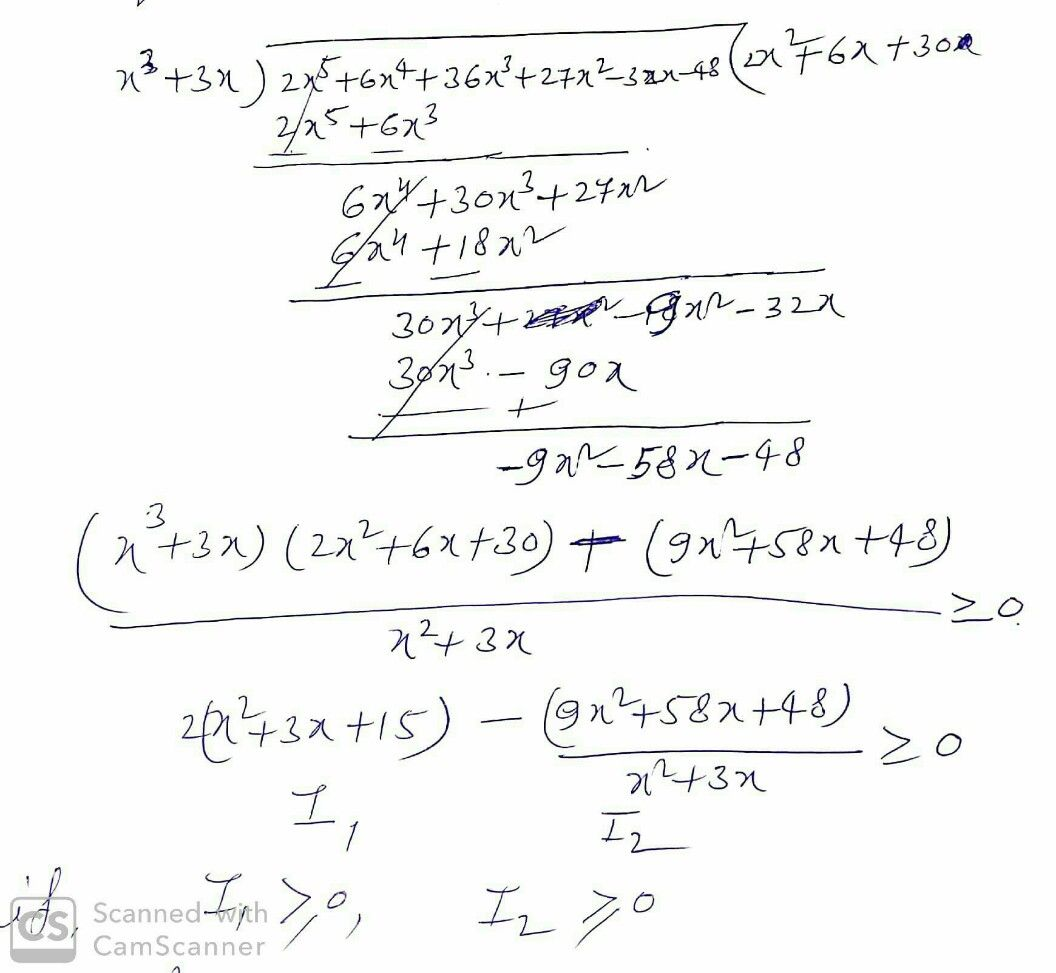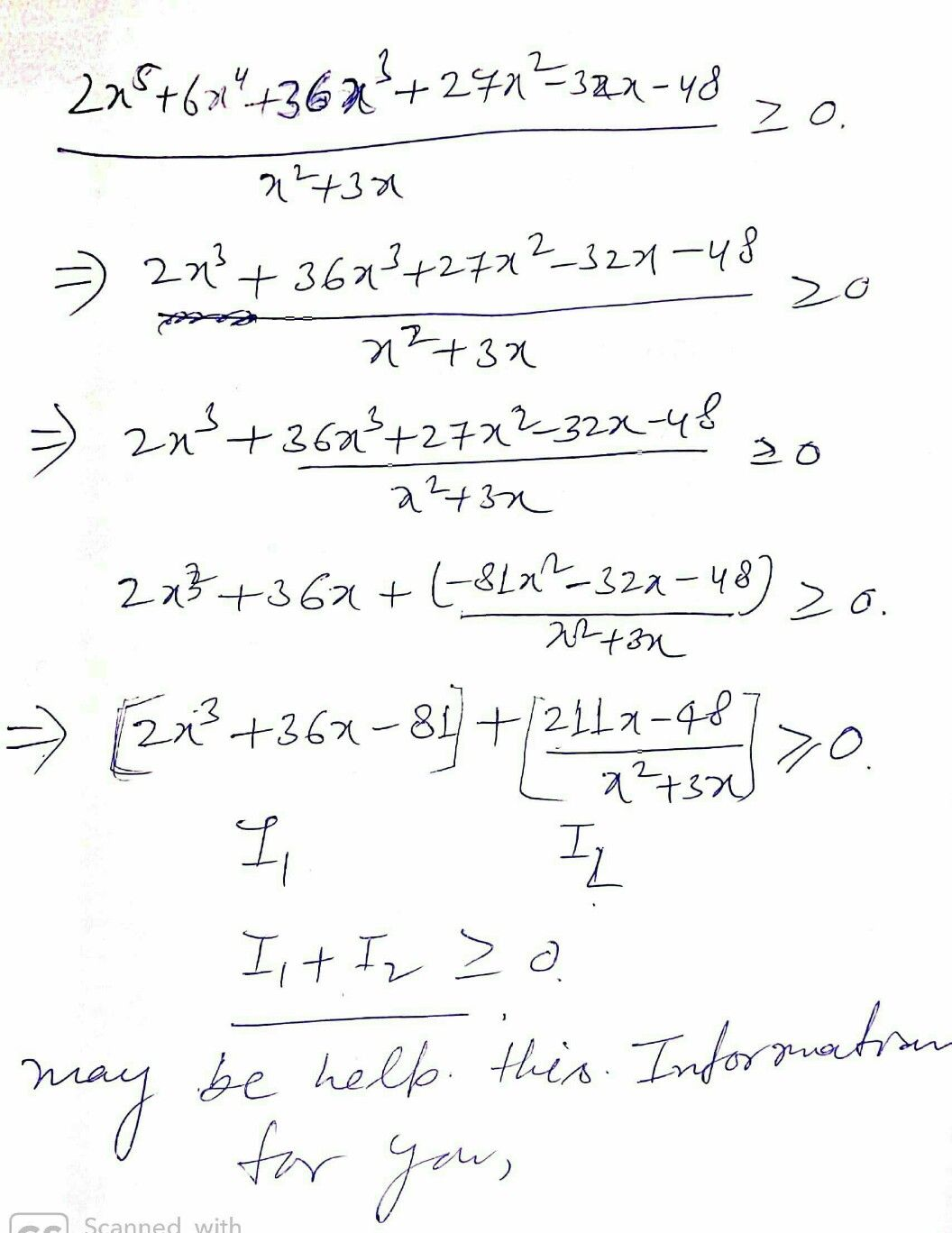Symbol
Problem$2x^{5}+6x^{2}+36x^{3}+23x^{2}-$ $32x-n8$ $x^{2-}$ $+$ $3$ $x$ Solve $8$ $x$